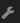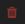Data attributes

# Data attributes

Once data is imported in PBI VizEdit, you need to select type of series you want to add to your visual in Data Attributes. This can be done via following menu:As soon as you click one of the buttons, it:

1. adds a series in the Data Attributes pane. You bind data with different attributes of the series here and also provide the kind of plot you want to have for this series.

2. adds a plot in the Visual Attributes pane. You update the overall appearance of the series data-points here.

You can add as many series as you want to visualize into the visual. You can change series type form theand remove the series by clickingbutton.

Attributes which can be bound with data are different for each series type as defined below:

## Bar series

using bar series one can place lines, horizontal bars (bar) or vertical bars (columns) on the visual. Following data attributes can be bound for bar:

• X Axis is plotted on bound horizontal axis. For horizontal bars, this is generally some numerical data and for vertical bars (columns) this is generally some categorical data.
• Y Axis is plotted on bound vertical axis. For horizontal bars, this is generally some categorical data and for vertical bars (columns) this is generally some numerical data.
• Bar label is the data which should be shown on each bar/column.
• Tooltip is used to show Tooltip (or hover text) for each bar/column.
• Bar width defines width of each bar/column. A value of 1 means bar/column shall assume all the width that is alloted to it, if there would have no width provided.
• Bar base defines from where the bar/column should start. For horizontal bars, it defines the distance of bar from vertical axis. For Vertical bars it is the distance of bar from horizontal axis.

## Bubble series

using bubble series one can place points (circles, squares, stars, etc.) on the visual. Following data attributes can be bound for bubble:

• X Axis is plotted on bound horizontal axis.
• Y Axis is plotted on bound vertical axis.
• Tooltip is used to show Tooltip (or hover text) for each point.
• Size is used to define the size of each point.

## Line series

using line series one can create line charts on the visual. These data attributes can be bound for line charts:

• X Axis is plotted on bound horizontal axis.
• Y Axis is plotted on bound vertical axis.
• Tooltip is used to show Tooltip (or hover text) for each line point.

## Area series

using area series one can create area charts on the visual. These data attributes can be bound for area charts:

• X Axis is plotted on bound horizontal axis.
• Y Axis is plotted on bound vertical axis.
• Tooltip is used to show Tooltip (or hover text) for each area point.

## Pie series

using pie series one can create a pie/donut charts on the visual. These data attributes can be bound for pie charts:

• X Axis defines the attributes against which data is to be plotted.
• Y Axis defines the size of pie.
• Tooltip is used to show Tooltip (or hover text) for pie.